# Approximation Question With Solution: Part 6

1. What approximate value should come in the place of the question (?) in the following question?A. 5120
B. 4800
C. 7200
D. 6400
E. 9600

2. What approximate value should come in the place of the question (?) in the following question?A. 24
B. 22
C. 28
D. 26
E. 18

3. What approximate value should come in the place of the question (?) in the following question?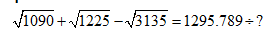A. 102
B. 108
C. 118
D. 104
E. 112

4. What approximate value should come in the place of the question (?) in the following question?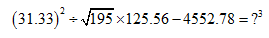A. 16
B. 22
C. 26
D. 6
E. 36

5. What approximate value should come in the place of the question (?) in the following question?A. 54
B. 216
C. 256
D. 196
E. 512

#### 1. Ans. D.

Solution:#### 2. Ans. C.

Solution: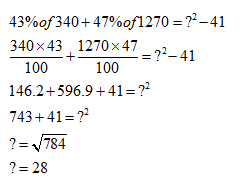#### 3. Ans. B.

Solution: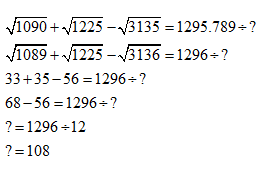#### 4. Ans. A.

Solution: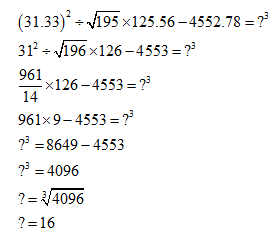#### 5. Ans. E.

Solution: